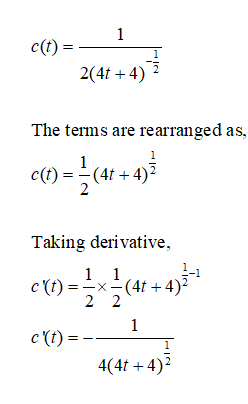# To test an individual's use of certain mineral, a researcher injects a small amount of radioactive form of that mineral into the persons bloodstream. Supposed to amount of the mineral remaining in the bloodstream t days after the initial injection is approximately C(t)= 1/2(4t+4)^-1/2. Fune the rate of change of the mineral level with respect to time for 0 days 8' milligrams per cubic centimeter. I tried to find the derivative of the formula and it equaled zero which didn't really make sense to me so I'm asking for help

Question
1 views

To test an individual's use of certain mineral, a researcher injects a small amount of radioactive form of that mineral into the persons bloodstream. Supposed to amount of the mineral remaining in the bloodstream t days after the initial injection is approximately C(t)= 1/2(4t+4)^-1/2. Fune the rate of change of the mineral level with respect to time for 0 days 8' milligrams per cubic centimeter.

I tried to find the derivative of the formula and it equaled zero which didn't really make sense to me so I'm asking for help

check_circle

Step 1

Hi, the term (4t+4)^-1/2 is unclear whether is present in the numerator or the denominator.

We are assuming it is in the denominator. The calculations are as per this assumption. In case the term is to be on the numerator, the calculations will change accordingly.

Step 2...

### Want to see the full answer?

See Solution

#### Want to see this answer and more?

Solutions are written by subject experts who are available 24/7. Questions are typically answered within 1 hour.*

See Solution
*Response times may vary by subject and question.
Tagged in